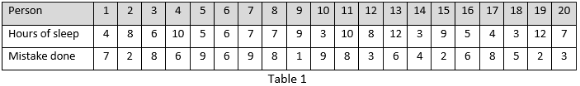May 18, 2023
• A psychologist studied the number of hours a worker sleeps at night and the number of errors made in a given task the following morning. Table 1 shows the results for the twenty people who were studied. It was also discovered that the average number of errors made by a normal person is 5.6.Based on Table 1 above:

• Illustrate the two variables given (separately) by means of a pictorial representation and comment on it.
• Calculate its measures of central tendency and measures of dispersion.
• State the main three measures of central tendencies and give appropriate examples to explain their advantages and disadvantages.
• Build a hypothesis that should use a t-test analysis using the five steps of hypothesis testing. Learners must complete steps 1-5 of hypothesis testing and show calculations made. Report the results as a research article would. Topic 7 t-test
• Calculate the correlation coefficient, r, and interpret the value.